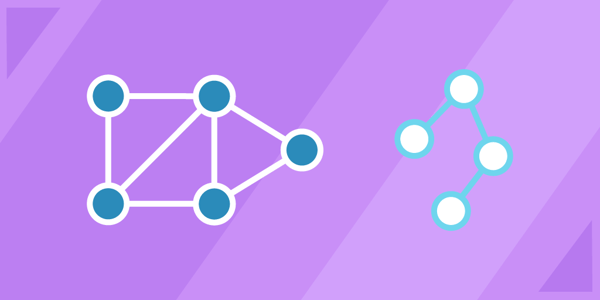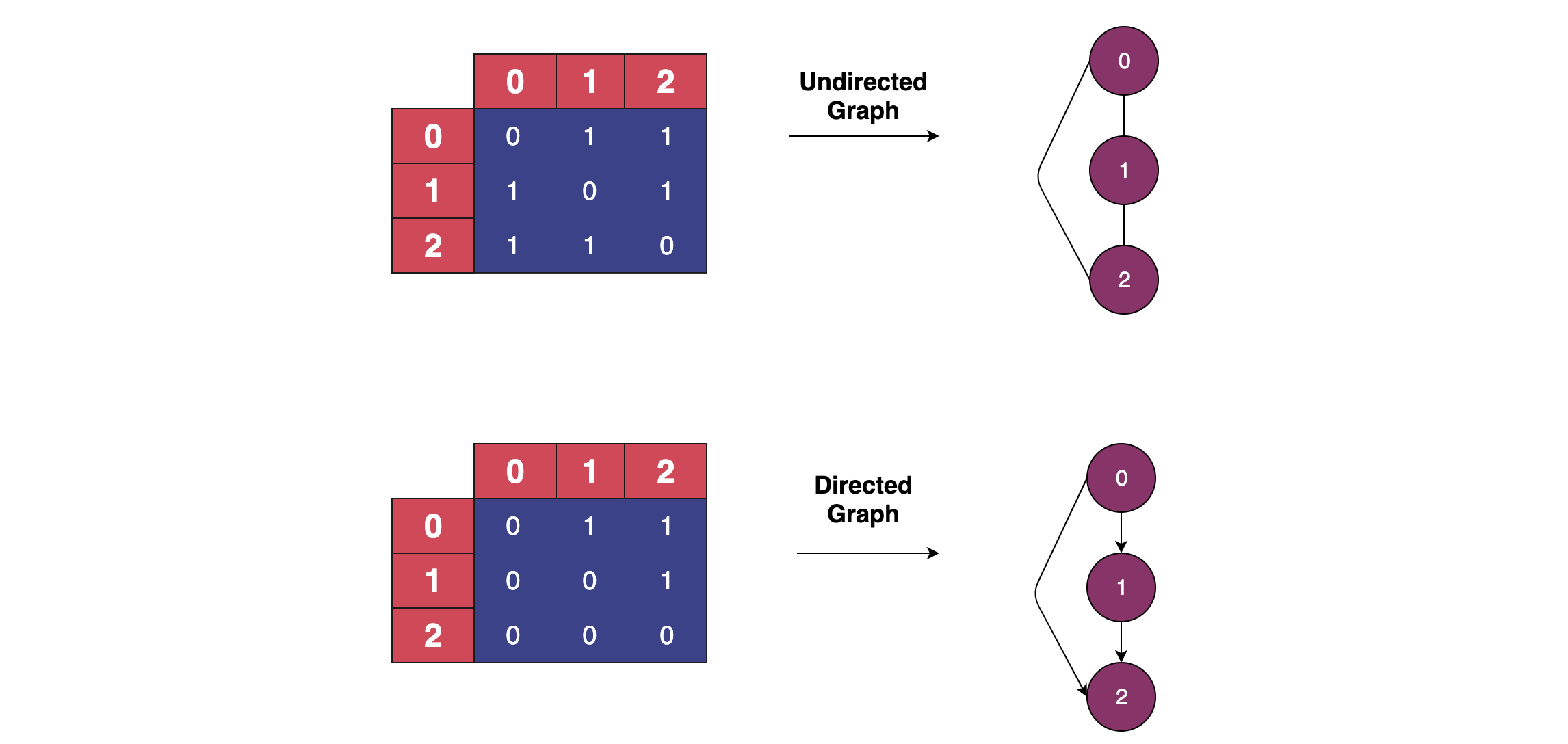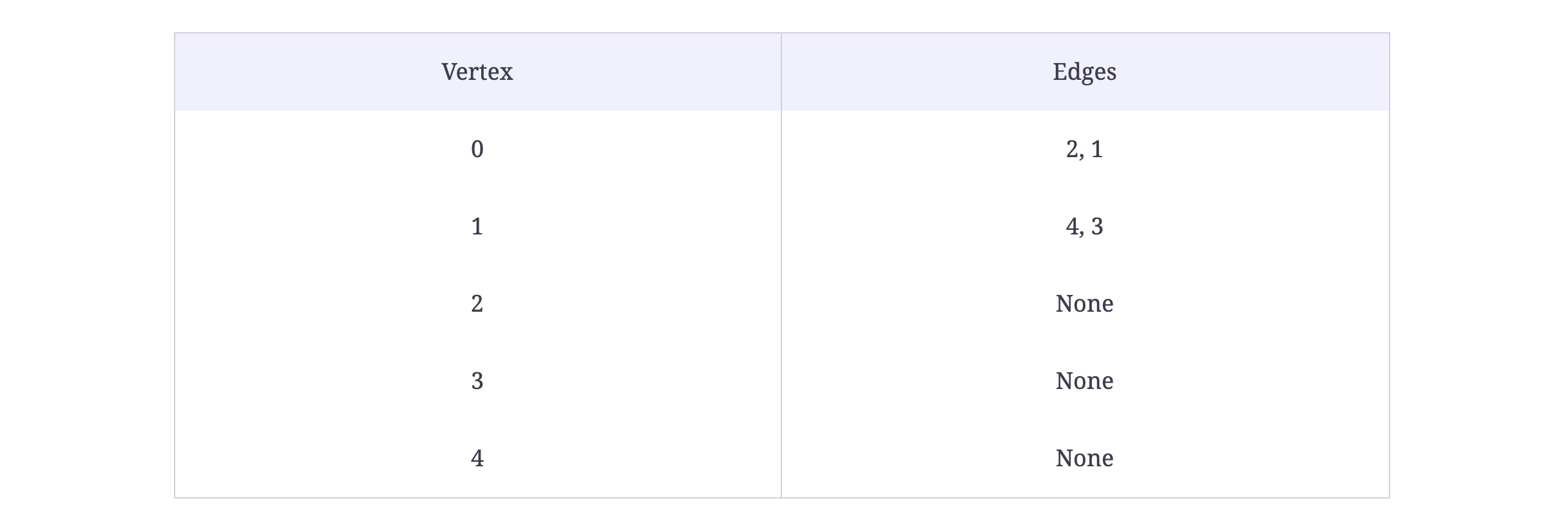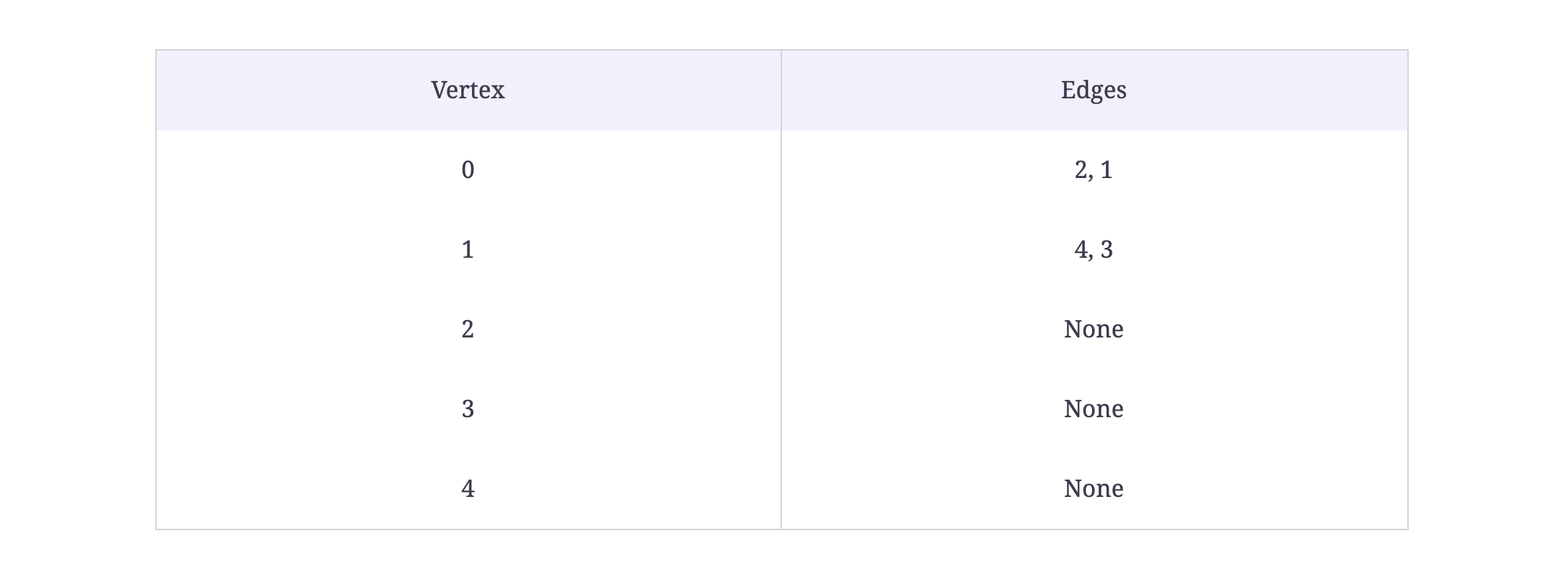# Algorithms 101: How to use graph algorithms

Dec 17, 2020 - 11 min readJerry EjonaviAlgorithms are one of the most common themes in coding interviews. In order to gain an advantage in interviews, it is important to be very familiar with the top algorithms and their implementations.

In today’s tutorial, we will be exploring graph algorithms. We’ll begin with an introduction to graph theory and graph algorithms. Next, we will learn how to implement a graph. Finally, we will examine common graph problems you can expect to see in a coding interview.

Today, we will learn:

## What are graph algorithms?

An algorithm is a mathematical process to solve a problem using a well-defined or optimal number of steps. It is simply the basic technique used to get a specific job done.

A graph is an abstract notation used to represent the connection between all pairs of objects. Graphs are widely-used mathematical structures visualized by two basic components: nodes and edges.Graph algorithms are used to solve the problems of representing graphs as networks like airline flights, how the Internet is connected, or social network connectivity on Facebook. They are also popular in NLP and machine learning to form networks.

Some of the top graph algorithms include:

• Implement depth-first traversal
• Calculate the number of nodes in a graph level
• Find all paths between two nodes
• Find all connected components of a graph
• Dijkstra’s algorithm to find shortest path in graph data
• Remove an edge

While graphs form an integral part of discrete mathematics, they also have practical uses in computer science and programming, including the following:

• Caller-callee relationships in a computer program represented as a graph
• The link structure of a website could be represented by a directed graph
• Neural networks are implemented using

## Properties of a graph

A graph, denoted by G, is represented by a set of vertices (V) or nodes linked at edges (E). The number of edges you have depends on the vertices. The edges may be directed or undirected.

In a directed graph, the nodes are linked in one direction. The edges here show a one-way relationship.

In an undirected graph, the edges are bi-directional, showing a two-way relationship.

Example: A good use-case of an undirected graph is Facebook friend suggestions algorithm. The user (node) has an edge running to a friend A (another node) who is in turn connected (or has an edge running) to friend B. Friend B is then suggested to the user.There are many other complex types of graphs that fall into different subsets. A directed graphs, for example, has strongly connected components when every vertex is reachable from every other vertex.

### Vertex

A vertex is a point where multiple lines meet. It is also called a node.

### Edge

An edge is a mathematical term used for a line that connects two vertices. Many edges can be formed from a single vertex. However, without a vertex, an edge cannot be formed. T​here must be a starting and ending vertex for each edge.

### Path

A path in a graph $G = (V,E)$ is a sequence of vertices v1, v2, …, vk, with the property that there are edges between $vi$ and $vi+1$. We say that the path goes from $v1$ to $vk$.

The sequence 6,4,5,1,26,4,5,1,2 defines a path from node 6 to node 2.

Similarly, other paths can be created by traversing the edges of the graph. A path is simple, if its vertices are all different.

### Walk

Walks are paths, but they don’t require a sequence of distinct vertices.

### Connected graph

A graph is connected if for every pair of vertices $u$ and $v$, there is a path from $u$ to $v$.

### Cycle

A cycle is a path v1, v2, …, vk for which the following are true:

• $k>2k>2$
• The first $k−1$ vertices are all different
• $v1=vk$

### Tree

A tree is a connected graph that does not contain a cycle.

### Loop

In a graph, if an edge is drawn from the vertex to itself, it is called a loop. In the illustration, V is a vertex whose edge, (V, V), is forming a loop.## How to represent graphs in code

Before we move on to solving problems using graph algorithms, it is important to first know how to represent graphs in code. Graphs can be represented as an adjacency matrix or adjacency list.

An adjacency matrix is a square matrix labeled by graph vertices and is used to represent a finite graph. The entries of the matrix indicate whether the vertex pair is adjacent or not in the graph.

In the adjacency matrix representation, you will need to iterate through all the nodes to identify a node’s neighbors.

  a b c d e
a 1 1 - - -
b - - 1 - -
c - - - 1 -
d - 1 1 - -


An adjacency list is used to represent a finite graph. The adjacency list representation allows you to iterate through the neighbors of a node easily. Each index in the list represents the vertex, and each node that is linked with that index represents its neighboring vertices.

1 a -> { a b }
2 b -> { c }
3 c -> { d }
4 d -> { b c }


For the base graph class below, we will be using the Adjacency List implementation as it performs faster for the algorithm solutions later in this article.

### The Graph Class

The requirements of our graph implementation are fairly straightforward. We would need two data members: the total number of vertices in the graph and a list to store adjacent vertices. We also need a method to add edges or a set of edges.

class AdjNode:
"""
A class to represent the adjacency list of the node
"""

def __init__(self, data):
"""
Constructor
:param data : vertex
"""
self.vertex = data
self.next = None

class Graph:
"""
"""

def __init__(self, vertices):
"""
Constructor
:param vertices : Total vertices in a graph
"""
self.V = vertices
self.graph = [None] * self.V

# Function to add an edge in an undirected graph

"""
:param source: Source Vertex
:param destination: Destination Vertex
"""

# Adding the node to the source node
node.next = self.graph[source]
self.graph[source] = node

# Adding the source node to the destination if undirected graph

# Intentionally commented the lines
#node.next = self.graph[destination]
#self.graph[destination] = node

def print_graph(self):
"""
A function to print a graph
"""
for i in range(self.V):
temp = self.graph[i]
while temp:
print(" -> {}".format(temp.vertex), end="")
temp = temp.next
print(" \n")

# Main program
if __name__ == "__main__":

V = 5  # Total vertices
g = Graph(V)

g.print_graph()

In the above example, we see the Python graph class. We’ve laid down the foundation of our graph class. The variable V contains an integer specifying the total number of vertices.

## Keep the learning going.

Prepare for Python interviews without scrubbing through videos or documentation. Educative’s text-based courses are easy to skim and feature live coding environments, making learning quick and efficient.

Ace the Python Coding Interview

## How to implement breadth-first traversal

Given a graph represented as an adjacency list and a starting vertex, your code should output a string containing the vertices of the graph listed in the correct order of traversal. As you traverse the graph from the starting vertex, you are to print each node’s right child first, then the left.

To solve this problem, the previously implemented Graph class is already prepended.

Input: A graph represented as an adjacency list and a starting vertex

Output: A string containing the vertices of the graph listed in the correct order of traversalSample Output:

result = "02143"
or
result = "01234"


Take a look and design a step-by-step algorithm before jumping on to the implementation. Try to solve it on your own first. If you get stuck, you can always refer to the solution provided in the solution section.

def bfs(graph, source):
"""
Function to print a BFS of graph
:param graph: The graph
:param source: starting vertex
:return:
"""

pass

### Solution

def bfs(my_graph, source):
"""
Function to print a BFS of graph
:param graph: The graph
:param source: starting vertex
:return:
"""

# Mark all the vertices as not visited
visited = [False] * (len(my_graph.graph))

# Create a queue for BFS
queue = []

# Result string
result = ""

# Mark the source node as
# visited and enqueue it
queue.append(source)
visited[source] = True

while queue:

# Dequeue a vertex from
# queue and print it
source = queue.pop(0)
result += str(source)

# Get all adjacent vertices of the
# dequeued vertex source. If a adjacent
# has not been visited, then mark it
# visited and enqueue it
while my_graph.graph[source] is not None:
data = my_graph.graph[source].vertex
if not visited[data]:
queue.append(data)
visited[data] = True
my_graph.graph[source] = my_graph.graph[source].next

return result

# Main to test the above program
if __name__ == "__main__":

V = 5
g = Graph(V)

print(bfs(g, 0))

We begin from a selected node and traverse the graph by layers. All neighbor nodes are explored. Then, we traverse we the next level. We traverse the graph horizontally, aka by each layer.

A graph may contain cycles. To avoid processing the same node again, we can use a boolean array that marks visited arrays. You can use a queue to store the node and mark it as visited. The queue should follow the First In First Out (FIFO) queuing method.

## How to implement depth-first traversal

In this problem, you have to implement the depth-first traversal. To solve this problem, the previously implemented graph class is already provided.

Input: A graph represented as an adjacency list and a starting vertex

Output: A string containing the vertices of the graph listed in the correct order of traversalSample Output:

result = "01342"
or
result = "02143"


Take a look and design a step-by-step algorithm before jumping on to the implementation. Try to solve it on your own first. If you get stuck, you can always refer to the solution provided in the solution section.

def dfs(graph, source):
"""
Function to print a DFS of graph
:param graph: The graph
:param source: starting vertex
:return:
"""

pass

### Solution

def dfs(my_graph, source):
"""
Function to print a DFS of graph
:param graph: The graph
:param source: starting vertex
:return: returns the traversal in a string
"""

# Mark all the vertices as not visited
visited = [False] * (len(my_graph.graph))

# Create a stack for DFS
stack = []

# Result string
result = ""

# Push the source
stack.append(source)

while stack:

# Pop a vertex from stack
source = stack.pop()

if not visited[source]:
result += str(source)
visited[source] = True

# Get all adjacent vertices of the popped vertex source.
# If a adjacent has not been visited, then push it
while my_graph.graph[source] is not None:
data = my_graph.graph[source].vertex
if not visited[data]:
stack.append(data)
my_graph.graph[source] = my_graph.graph[source].next

return result

# Main to test the above program
if __name__ == "__main__":

V = 5
g = Graph(V)

print(dfs(g, 0))

The depth-first graph algorithm uses the idea of backtracking. Here, ‘backtrack’ means to move forward as long as there are no more nodes in the current path, then to move backward on the same path to find nodes to traverse.

## How to remove an edge

In this problem, you must implement the remove_edge function which takes a source and a destination as arguments. If an edge exists between the two, it should be deleted.

Input: A graph, a source (integer), and a destination (integer)

Output: A BFS traversal of the graph with the edge between the source and the destination removed

First, take a close look at this problem and design a step-by-step algorithm before jumping to the implementation. Try it yourself before checking the solution!

def remove_edge(graph, source, destination):
"""
A function to remove an edge
:param graph: A graph
:param source: Source Vertex
:param destination: Destination Vertex
"""

pass

### Solution

This challenge is very similar to the deletion in the linked list, if you are familiar with it.

Our vertices are stored in a linked list. First, we access the source linked list. If the head node of the source linked list holds the key to be deleted, we shift the head one step forward and return the graph.

If the key to be deleted is in the middle of the linked list, we keep track of the previous node and connect the previous node with the next node when the destination encounters.

## More interview questions

Below are other interview questions that you can try your hand at solving:

• Check if a graph is strongly connected.
• Count all possible paths between two vertices.
• Count the number of nodes at a given level in a tree using breadth-first search (BFS)
• Count the number of trees in a forest.
• Detect a cycle in a graph.
• Find a Mother Vertex in a graph.
• Find K cores of an undirected graph.
• Find the path in a rectangle with circles.
• Use a minimum spanning tree to design network
• Find the shortest path to reach one prime to others by changing a single digit at a time.
• Find the smallest binary digit multiple of given number.
• Height of a generic tree from parent array.
• Iterative Depth First Search(DFS).
• Use Kruskal’s algorithm to find a minimum spanning forest of an undirected edge-weighted graph
• Use Prim’s Minimum Spanning Tree Greedy algorithm (MST)
• Minimum initial vertices to traverse whole matrix with given conditions.
• Use Bellman–Ford algorithm to compute the shortest paths from a vertex to other vertices in a weighted graph
• Transpose a graph.
• Water Jug problem using BFS.

## What to learn next

Congratulations on making it to the end. You should know understand graphs in Python and understand what to prepare for graph-related coding interview questions.

If you’d like to learn more about algorithms in Python, check out Educative’s learning path Ace the Python Coding Interview. In these modules, you’ll brush up on data structures, algorithms, and important syntax by practicing hundreds of real interview questions

By the end, you’ll even be able to confidently answer multithreading and concurrency questions.

Happy learning!